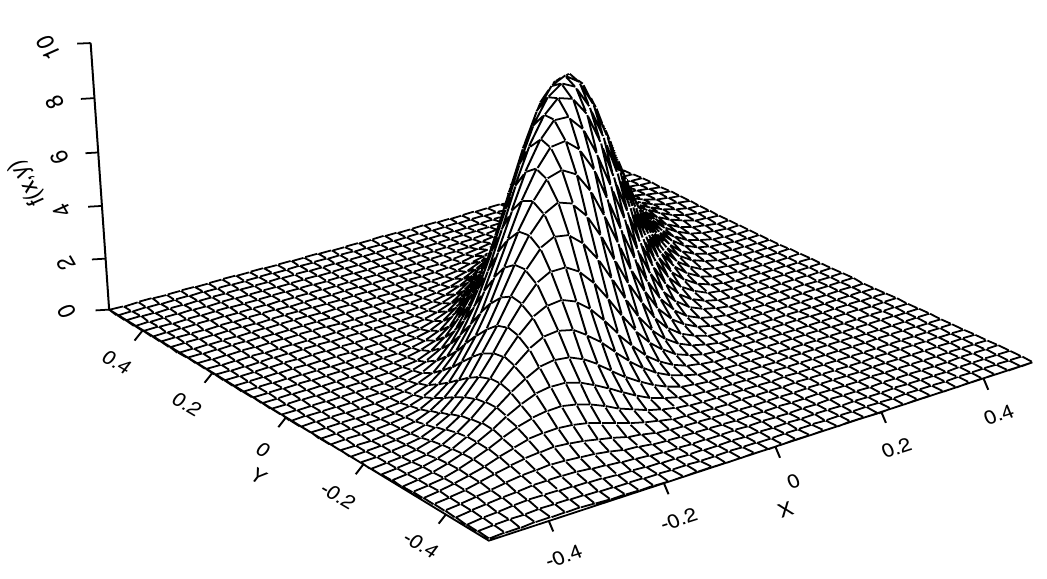# Multivariate distributions part I

## Instructions:

Use the left and right arrow keys to navigate the presentation forward and backward respectively. You can also use the arrows at the bottom right of the screen to navigate with a mouse.

FAIR USE ACT DISCLAIMER:
This site is for educational purposes only. This website may contain copyrighted material, the use of which has not been specifically authorized by the copyright holders. The material is made available on this website as a way to advance teaching, and copyright-protected materials are used to the extent necessary to make this class function in a distance learning environment. The Fair Use Copyright Disclaimer is under section 107 of the Copyright Act of 1976, allowance is made for “fair use” for purposes such as criticism, comment, news reporting, teaching, scholarship, education and research.

## Outline

• The following topics will be covered in this lecture:
• The distribution function of a random vector
• The density function of a random vector
• Marginal versus conditional probability
• Expected value

## Introducing multiple random variables

• We have now learned about how to describe behavior of a single random variable and the mathematical structure of how to think about multiple variables in matrices and functions.

• We will now introduce the basic tools of statistics and probability theory for multivariate analysis;

• we will be studying the relations between $$p$$ random variables that will often covary together in their conditional probabilities.
• To begin with we will make the extension of random variables to random vectors, using our understanding of basic matrix theory.

• Some notions like the expected / center of mass will translate directly over linear combinations of random variables.

• Recall, for random variables $$X,Y$$ and a constant scalars $$a,b$$ we have $\mathbb{E}\left[ a X + b Y\right] = a \mathbb{E}\left[X\right] + b \mathbb{E}\left[Y\right].$

• The same idea extends algebraicly for random vectors $$\boldsymbol{\xi}_1, \boldsymbol{\xi}_2$$ and constant matrices $$\mathbf{A},\mathbf{B}$$, we can write $\mathbb{E}\left[\mathbf{A}\boldsymbol{\xi}_1 + \mathbf{B}\boldsymbol{\xi}_2 \right] = \mathbf{A}\mathbb{E}\left[ \boldsymbol{\xi}_1\right] + \mathbf{B}\mathbb{E}\left[\boldsymbol{\xi}_2\right].$

• While the concept of the center of mass remains basically the same, we will need to make some additional considerations when we measure the spread of random variables and how they relate to others.

## The cumulitive distribution function

• We will begin our consideration in $$p=2$$ dimensions, as all properties described in the following will extend (with minor modifications) to arbitrarily large but finite $$p$$.

• Let the vector $$\boldsymbol{\xi}$$ be defined as

\begin{align} \boldsymbol{\xi} = \begin{pmatrix} \xi_1 \\ \xi_2 \end{pmatrix} \end{align} where each of the above components $$\xi_i$$ is a rv.

• We can define the cumulative distribution function in a similar way to the definition in one variable.

• Let $$x_1,x_2$$ be two fixed real values forming a constant vector as

$\mathbf{x} = \begin{pmatrix} x_1 \\ x_2\end{pmatrix}.$

• Define the comparison operator between two vectors $$\mathbf{y}, \mathbf{x}$$ as

$\mathbf{y} \leq \mathbf{x} \Leftrightarrow y_i \leq x_i \text{ for each and every }i$

• The cumulative distribution function $$F_\boldsymbol{\xi}$$, describing the probability of realizations of $$\boldsymbol{\xi}$$, is thus given as,

$F_\boldsymbol{\xi}(\mathbf{x}) = P(\boldsymbol{\xi}\leq \mathbf{x} ) = P(\xi_i \leq x_i \text{ for each and every }i)$

### The joint probability density function

• Recall that the cdf

\begin{align} F_\boldsymbol{\xi}:\mathbb{R}^2 & \rightarrow [0,1] \\ \mathbf{x} & \rightarrow P(\boldsymbol{\xi}\leq \mathbf{x}) \end{align} is a function of the variables $$(x_1,x_2)$$.

• Suppose then that $$F_\boldsymbol{\xi}$$ has continuous second partial derivatives in $$\partial_{x_1} \partial_{x_2}F_\boldsymbol{\xi} = \partial_{x_2}\partial_{x_1}F_\boldsymbol{\xi}$$.

• We then can take the probability density function $$f$$ to be defined as \begin{align} f_\boldsymbol{\xi}:\mathbb{R}^2 & \rightarrow \mathbb{R}\\ \mathbf{x} &\rightarrow \partial_{x_1}\partial_{x_2}F_\boldsymbol{\xi}(\mathbf{x}) \end{align}

• In the above definition, we have constructed the density function in the same way as in one variable;

• specifically, in the case where $$f_\boldsymbol{\xi}$$ itself is differentiable, we have defined the cdf as the anti-derivative of the density:

\begin{align} F_\boldsymbol{\xi}(\mathbf{x}) = \int_{-\infty}^{x_1} \int_{-\infty}^{x_2} f_\boldsymbol{\xi}(s_1, s_2) \mathrm{d}s_1 \mathrm{d}s_2 \end{align}

### The joint probability density function

• Recall that we defined the relationship between the cdf and the density as

\begin{align} F_\boldsymbol{\xi}(\mathbf{x}) = \int_{-\infty}^{x_1} \int_{-\infty}^{x_2} f_\boldsymbol{\xi}(s_1, s_2) \mathrm{d}s_1 \mathrm{d}s_2 \end{align}

• By the above definition, we have to have that

\begin{align} P(- \infty < \boldsymbol{\xi} < \infty) = \int_{-\infty}^{\infty} \int_{-\infty}^{\infty} f_\boldsymbol{\xi}(s_1, s_2) \mathrm{d}s_1 \mathrm{d}s_2 = 1 \end{align}

• We note that, as usual, the density function $$f$$ must always be positive as the cdf $$F_\boldsymbol{\xi}$$ is everywhere increasing for a positive increase in $$x_1,x_2$$.

• Note, if we defined this over $$p\geq 2$$ variables, all of the above extends identically when $$F_\boldsymbol{\xi}$$ has derivatives defined in arbitrary arrangements of the $$p$$-th partial derivatives in each $$\partial_{x_i}$$.

• We then construct $$F_\boldsymbol{\xi}$$ once again as the anti-derivative of $$f_\boldsymbol{\xi}$$ in all univariate partial derivatives.

### The joint probability density function

• Partiuclarly, we will again view the density function like the curve of the univariate case, but for two variables we see this as a surface above the $$x_1,x_2$$ plane.Courtesy of: Dekking, et al. A Modern Introduction to Probability and Statistics. Springer Science & Business Media, 2005.

• To the right, we see the multivariate Gaussian bell surface that defines the multivariate normal distribution in two variables.
• In one variable, the probability $$P(\xi_1 \leq x_1)$$ was associated to the area under the curve, computed by the integral of the density.